Demo problem: Bending of a cantilever beam

In this example we solve a classical solid mechanics problem, the bending of a cantilever beam, subject to a pressure loading on its upper face and/or gravity. We assume that the material behaves like a generalised Hookean solid with elastic modulus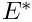and Poisson's ratio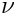Here is a sketch: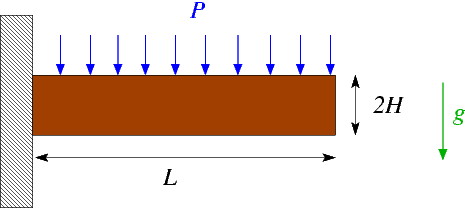Sketch of the problem.

This problem is interesting because it has an (approximate) St. Venant solution for the stress field which may be constructed in terms of an Airy stress function (see, e.g. H. Eschenauer & W. Schnell "Elastizitaetstheorie I", BI Wissenschaftsverlag, 2nd edition, 1986).

# Results

The following figure shows an animation of beam's deformation in response to an increase in the uniform pressure,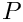, at zero gravity,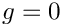. The colour contours represent the magnitude of the "horizontal" component of the 2nd Piola-Kirchhoff stress tensor,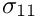. Its approximately linear variation across the beam's thickness indicates a bending-type stress distribution with the stress being positive (tensile) at the top and negative (compressive) at the bottom.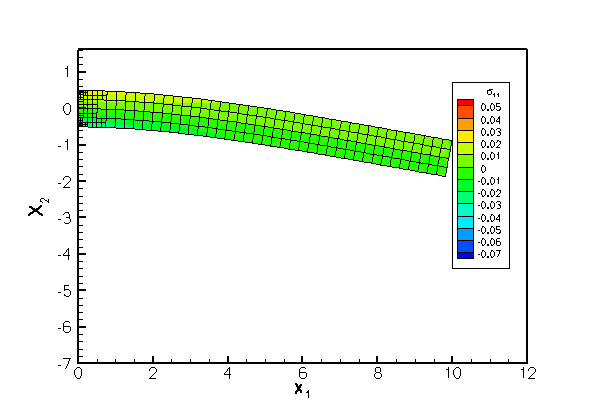Animation of the beam's deformation with contours of its horizontal' stress component.

The next figure shows a comparison of the computational predictions for(in green), and the approximate analytical solution (in red).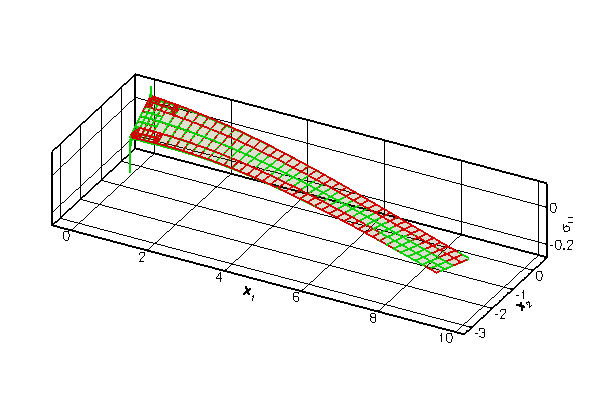Comparison of the computed distribution of the horizontal' stress (in green) against the approximate analytical solution (in red).

The agreement between the two solutions is excellent over most of the domain, apart from two small regions near the left end of the beam where stress singularities develop at the vertices of the domain. The singularities arise because the zero-tangential stress boundary condition on the top and bottom faces is inconsistent with the zero-displacement boundary condition on the left face. The singularities are not captured by the approximate analytical solution. oomph-lib's automatic mesh adaptation refines the mesh in an attempt to capture the rapid variations of the stresses in these regions.

# Global parameters and functions

As usual, we define a namespace, Global_Physical_Variables, to define the problem parameters: the dimensions of the cantilever beam, (a pointer to) a constitutive equation, and its parameters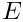and.

//=======start_namespace==========================================
/// Global variables
//================================================================
{
/// Half height of beam
double H=0.5;
/// Length of beam
double L=10.0;
/// Pointer to constitutive law
ConstitutiveLaw* Constitutive_law_pt;
/// Elastic modulus
double E=1.0;
/// Poisson's ratio
double Nu=0.3;
//////////////////////////////////////////////////////////////////// ////////////////////////////////...
double E
Elastic modulus.
double L
Length of beam.
double Nu
Poisson's ratio.
ConstitutiveLaw * Constitutive_law_pt
Pointer to constitutive law.
double H
Half height of beam.

We refer to the document "Solid mechanics: Theory and implementation" for a detailed discussion of the non-dimensionalisation and merely recall that by setting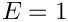, we imply that all stresses are non-dimensionalised with the structure's dimensional Young's modulus. Similarly, by setting the half-thickness of the beam to 0.5, we imply that the beam's dimensional thickness,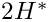is used to non-dimensionalise all lengths.

Next, we define a function that defines the constant pressure load on the upper face of the cantilever,

/// Uniform pressure
double P = 0.0;
/// Constant pressure load. The arguments to this function are imposed
/// on us by the SolidTractionElements which allow the traction to
/// depend on the Lagrangian and Eulerian coordinates x and xi, and on the
/// outer unit normal to the surface. Here we only need the outer unit
/// normal.
void constant_pressure(const Vector<double> &xi, const Vector<double> &x,
const Vector<double> &n, Vector<double> &traction)
{
unsigned dim = traction.size();
for(unsigned i=0;i<dim;i++)
{
traction[i] = -P*n[i];
}
} // end traction
void constant_pressure(const Vector< double > &xi, const Vector< double > &x, const Vector< double > &n, Vector< double > &traction)
Constant pressure load. The arguments to this function are imposed on us by the SolidTractionElements...
double P
Uniform pressure.

and a gravitational body force, acting in the negative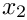-direction,

/// Non-dim gravity
double Gravity=0.0;
/// Non-dimensional gravity as body force
void gravity(const double& time,
const Vector<double> &xi,
Vector<double> &b)
{
b=0.0;
b=-Gravity;
}
} //end namespace
void gravity(const double &time, const Vector< double > &xi, Vector< double > &b)
Non-dimensional gravity as body force.
double Gravity
Non-dim gravity.

# The driver code

The driver code is very short. We start by building a GeneralisedHookean constitutive equation object and store a pointer to it in the namespace Global_Physical_Variables. Next we construct the problem object, using (a wrapped version of) oomph-lib's RefineableQPVDElement<2,3> – a nine-node quadrilateral displacement-based solid mechanics element. (The wrapper is used to change the element's output function; see Comment: Customising an element's output function for details).

//=======start_of_main==================================================
/// Driver for cantilever beam loaded by surface traction and/or
/// gravity
//======================================================================
int main()
{
// Create generalised Hookean constitutive equations
new GeneralisedHookean(&Global_Physical_Variables::Nu,
//Set up the problem
int main()
Driver for cantilever beam loaded by surface traction and/or gravity.
Problem class for the cantilever "beam" structure.

The subsequent lines may be uncommented to experiment with different element types as suggested in the Exercises.

// Uncomment these as an exercise
// CantileverProblem<MySolidElement<
// RefineableQPVDElementWithContinuousPressure<2> > > problem;
// CantileverProblem<MySolidElement<
// RefineableQPVDElementWithPressure<2> > > problem;

We initialise the load parameters and perform a parameter study in which we increment the pressure load in small steps. The gravitational body force remains switched off to allow the comparison with the analytical solution which only applies to the case with zero body force.

// Initial values for parameter values
// Max. number of adaptations per solve
//Parameter incrementation
unsigned nstep=5;
double p_increment=1.0e-5;
for(unsigned i=0;i<nstep;i++)
{
// Solve the problem with Newton's method, allowing
// Doc solution
problem.doc_solution();
}
} //end of main
void doc_solution()
Doc the solution.

# The problem class

The problem class contains the usual member functions, including separate access functions to the two sub-meshes: The "bulk" mesh that contains the 2D solid elements, and a separate mesh in which we store the 1D SolidTractionElements that apply the traction boundary condition on the beam's upper face. As usual, we remove these elements before adapting the bulk mesh and re-attach them afterwards, using the functions Problem::actions_before_adapt() and Problem::actions_after_adapt().

//=============begin_problem============================================
/// Problem class for the cantilever "beam" structure.
//======================================================================
template<class ELEMENT>
class CantileverProblem : public Problem
{
public:
/// Constructor:
/// Update function (empty)
/// Update function (empty)
/// Access function for the solid mesh
{return Solid_mesh_pt;}
/// Access function to the mesh of surface traction elements
SolidMesh*& traction_mesh_pt(){return Traction_mesh_pt;}
/// Actions before adapt: Wipe the mesh of traction elements
/// Actions after adapt: Rebuild the mesh of traction elements
/// Doc the solution
void doc_solution();
SolidMesh *& traction_mesh_pt()
Access function to the mesh of surface traction elements.
Pointer to solid mesh.
void actions_before_newton_solve()
Update function (empty)
void actions_after_newton_solve()
Update function (empty)
Actions before adapt: Wipe the mesh of traction elements.
SolidMesh * Traction_mesh_pt
Pointer to mesh of traction elements.
Access function for the solid mesh.
CantileverProblem()
Constructor:
Actions after adapt: Rebuild the mesh of traction elements.

The creation/deletion of the SolidTractionElements is performed by private helper functions. We also store a pointer to a node on the tip of the beam and will record its displacement as a function of the applied load in a trace file.

private:
/// Pass pointer to traction function to the
/// elements in the traction mesh
void set_traction_pt();
/// Create traction elements
void create_traction_elements();
/// Delete traction elements
void delete_traction_elements();
/// Trace file
ofstream Trace_file;
/// Pointers to node whose position we're tracing
Node* Trace_node_pt;
/// Pointer to solid mesh
/// Pointer to mesh of traction elements
SolidMesh* Traction_mesh_pt;
/// DocInfo object for output
DocInfo Doc_info;
};

# The problem constructor

The constructor builds the bulk mesh (the standard RefineableRectangularQuadMesh that we already used in many previous examples, upgraded to a SolidMesh, via the procedure discussed in the document Solid mechanics: Theory and implementation), using the element type specified by the template parameter and the dimensions specified in the namespace Global_Physical_Variables.

//===========start_of_constructor=======================================
/// Constructor:
//======================================================================
template<class ELEMENT>
{
// Create the mesh
// # of elements in x-direction
unsigned n_x=20;
// # of elements in y-direction
unsigned n_y=2;
// Domain length in x-direction
// Domain length in y-direction
// Shift mesh downwards so that centreline is at y=0:
Vector<double> origin(2);
origin=0.0;
origin=-0.5*l_y;
//Now create the mesh
n_x,n_y,l_x,l_y,origin);

We employ the Z2ErrorEstimator to assess the accuracy of the computed solution and to control the adaptive mesh refinement. When used with the RefineableQPVDElements, the Z2ErrorEstimator uses the components of Green's strain tensor as "fluxes" in its "flux recovery procedure".

// Set error estimator
solid_mesh_pt()->spatial_error_estimator_pt()=new Z2ErrorEstimator;

Next, we pass the constitutive equations and the gravitational body force to the elements, select a control node, and perform one uniform mesh refinement.

//Assign the physical properties to the elements before any refinement
//Loop over the elements in the main mesh
unsigned n_element =solid_mesh_pt()->nelement();
for(unsigned i=0;i<n_element;i++)
{
//Cast to a solid element
ELEMENT *el_pt = dynamic_cast<ELEMENT*>(solid_mesh_pt()->element_pt(i));
// Set the constitutive law
el_pt->constitutive_law_pt() =
//Set the body force
el_pt->body_force_fct_pt() = Global_Physical_Variables::gravity;
}
// Choose a control node: The last node in the solid mesh
unsigned nnod=solid_mesh_pt()->nnode();
Trace_node_pt=solid_mesh_pt()->node_pt(nnod-1);
// Refine the mesh uniformly
solid_mesh_pt()->refine_uniformly();

We create a new mesh for the SolidTractionElements and build the elements using the helper function create_traction_elements() before adding both submeshes to the problem and combining them into a global mesh.

// Construct the traction element mesh
Traction_mesh_pt=new SolidMesh;
create_traction_elements();
// Pass pointer to traction function to the elements
// in the traction mesh
set_traction_pt();
// Solid mesh is first sub-mesh
// Build combined "global" mesh
build_global_mesh();

We pin the position of all nodes on the left boundary (boundary 3) of the bulk mesh. The subsequent call to PVDEquationsBase<2>::pin_redundant_nodal_solid_pressures() is unnecessary (but harmless) for the solid elements used in this driver code since RefineableQPVDElements do not contain pressure degrees of freedom. It is a good idea to include this call anyway since our problem class is templated by the element type and may therefore also be used with other elements (see Exercises ; we refer to another tutorial for a detailed discussion of the need to pin "redundant" pressure degrees of freedom in computations with spatial adaptivity.)

// Pin the left boundary (boundary 3) in both directions
unsigned n_side = mesh_pt()->nboundary_node(3);
// Loop over the nodes
for(unsigned i=0;i<n_side;i++)
{
solid_mesh_pt()->boundary_node_pt(3,i)->pin_position(0);
solid_mesh_pt()->boundary_node_pt(3,i)->pin_position(1);
}
// Pin the redundant solid pressures (if any)
PVDEquationsBase<2>::pin_redundant_nodal_solid_pressures(
solid_mesh_pt()->element_pt());

Finally, we assign the equation numbers, create a DocInfo object and open a trace file in which we shall record the beam's load/displacement characteristics.

//Attach the boundary conditions to the mesh
cout << assign_eqn_numbers() << std::endl;
// Set output directory
Doc_info.set_directory("RESLT");
// Open trace file
char filename;
sprintf(filename,"%s/trace.dat",Doc_info.directory().c_str());
Trace_file.open(filename);
} //end of constructor

Following our usual procedure, we delete the SolidTractionElements before adapting the bulk mesh:

/// Actions before adapt: Wipe the mesh of traction elements
//========================================================================
template<class ELEMENT>
{
// Kill the traction elements and wipe surface mesh
delete_traction_elements();
// Rebuild the Problem's global mesh from its various sub-meshes
rebuild_global_mesh();

The SolidTractionElements are re-attached after the mesh adaptation. Again, the call to PVDEquationsBase<2>::pin_redundant_nodal_solid_pressures() is not strictly necessary for the elements used in the present driver code but is included "for safety".

/// Actions after adapt: Rebuild the mesh of traction elements
//========================================================================
template<class ELEMENT>
{
// Create traction elements from all elements that are
create_traction_elements();
// Rebuild the Problem's global mesh from its various sub-meshes
rebuild_global_mesh();
// Pin the redundant solid pressures (if any)
PVDEquationsBase<2>::pin_redundant_nodal_solid_pressures(
solid_mesh_pt()->element_pt());
// Set pointer to prescribed traction function for traction elements
set_traction_pt();

# Setting the pointer to the traction function

The helper function set_traction_pt() is used to pass the pointer to the traction function to the SolidTractionElements.

//==================start_of_set_traction_pt==============================
/// Set pointer to traction function for the relevant
/// elements in the traction mesh
//========================================================================
template<class ELEMENT>
{
// Loop over the elements in the traction element mesh
// for elements on the top boundary (boundary 2)
unsigned n_element=traction_mesh_pt()->nelement();
for(unsigned i=0;i<n_element;i++)
{
//Cast to a solid traction element
SolidTractionElement<ELEMENT> *el_pt =
dynamic_cast<SolidTractionElement<ELEMENT>*>
(traction_mesh_pt()->element_pt(i));
//Set the traction function
}
}// end of set traction pt
void set_traction_pt()
Pass pointer to traction function to the elements in the traction mesh.

# Creating the traction elements

The helper function create_traction_elements() is used to create the SolidTractionElements and to store them in the appropriate sub-mesh.

//============start_of_create_traction_elements==============================
/// Create traction elements
//=======================================================================
template<class ELEMENT>
{
// Traction elements are located on boundary 2:
unsigned b=2;
// How many bulk elements are adjacent to boundary b?
unsigned n_element = solid_mesh_pt()->nboundary_element(b);
// Loop over the bulk elements adjacent to boundary b
for(unsigned e=0;e<n_element;e++)
{
// Get pointer to the bulk element that is adjacent to boundary b
ELEMENT* bulk_elem_pt = dynamic_cast<ELEMENT*>(
solid_mesh_pt()->boundary_element_pt(b,e));
//Find the index of the face of element e along boundary b
int face_index = solid_mesh_pt()->face_index_at_boundary(b,e);
// Create new element and add to mesh
(bulk_elem_pt,face_index));
}
// Pass the pointer to the traction function to the traction elements
set_traction_pt();
} // end of create_traction_elements
void create_traction_elements()
Create traction elements.

# Deleting the traction elements

The helper function delete_traction_elements() is used to delete the SolidTractionElements.

//============start_of_delete_traction_elements==============================
/// Delete traction elements and wipe the traction meshes
//=======================================================================
template<class ELEMENT>
{
// How many surface elements are in the surface mesh
unsigned n_element = Traction_mesh_pt->nelement();
// Loop over the surface elements
for(unsigned e=0;e<n_element;e++)
{
// Kill surface element
delete Traction_mesh_pt->element_pt(e);
}
// Wipe the mesh
Traction_mesh_pt->flush_element_and_node_storage();
} // end of delete_traction_elements
void delete_traction_elements()
Delete traction elements.

# Post-processing

The post-processing function doc_solution() outputs the finite-element solution, using the modified output function defined in the wrapper class MySolidElement, discussed below.

//==============start_doc===========================================
/// Doc the solution
//==================================================================
template<class ELEMENT>
{
ofstream some_file;
char filename;
// Number of plot points
unsigned n_plot = 5;
// Output shape of and stress in deformed body
//--------------------------------------------
sprintf(filename,"%s/soln%i.dat",Doc_info.directory().c_str(),
Doc_info.number());
some_file.open(filename);
solid_mesh_pt()->output(some_file,n_plot);
some_file.close();

Next we output the approximate St. Venant solution at the same plot points and write the load/displacement characteristics to the trace file.

// Output St. Venant solution
//---------------------------
sprintf(filename,"%s/exact_soln%i.dat",Doc_info.directory().c_str(),
Doc_info.number());
some_file.open(filename);
// Element dimension
unsigned el_dim=2;
Vector<double> s(el_dim);
Vector<double> x(el_dim);
Vector<double> xi(el_dim);
DenseMatrix<double> sigma(el_dim,el_dim);
// Constants for exact (St. Venant) solution
double a=-1.0/4.0*Global_Physical_Variables::P;
double c=1.0/8.0*Global_Physical_Variables::P/
// Loop over all elements to plot exact solution for stresses
unsigned nel=solid_mesh_pt()->nelement();
for (unsigned e=0;e<nel;e++)
{
// Get pointer to element
SolidFiniteElement* el_pt=dynamic_cast<SolidFiniteElement*>(
solid_mesh_pt()->element_pt(e));
some_file << "ZONE I=" << n_plot << ", J=" << n_plot << std::endl;
//Loop over plot points
for(unsigned l2=0;l2<n_plot;l2++)
{
s = -1.0 + l2*2.0/(n_plot-1);
for(unsigned l1=0;l1<n_plot;l1++)
{
s = -1.0 + l1*2.0/(n_plot-1);
// Get Eulerian and Lagrangian coordinates
el_pt->interpolated_x(s,x);
el_pt->interpolated_xi(s,xi);
//Output the x,y,..
for(unsigned i=0;i<el_dim;i++)
{some_file << x[i] << " ";}
// Change orientation of coordinate system relative
// to solution in lecture notes
double yy=xi;
// Approximate analytical (St. Venant) solution
sigma(0,0)=c*(6.0*xx*xx*yy-4.0*yy*yy*yy)+
6.0*d*yy;
sigma(1,1)=2.0*(a+b*yy+c*yy*yy*yy);
sigma(1,0)=2.0*(b*xx+3.0*c*xx*yy*yy);
sigma(0,1)=sigma(1,0);
// Output stress
some_file << sigma(0,0) << " "
<< sigma(1,0) << " "
<< sigma(1,1) << " "
<< std::endl;
}
}
}
some_file.close();
// Write trace file: Load/displacement characteristics
Trace_file << Global_Physical_Variables::P << " "
<< Trace_node_pt->x(0) << " "
<< Trace_node_pt->x(1) << " "
<< std::endl;
// Increment label for output files
Doc_info.number()++;
} //end doc

## Exercises

1. Modify the driver code so that the cantilever beam is loaded by gravity rather than a pressure load. Consult the document "Solid mechanics: Theory and implementation" for details on the non-dimensionalisation of the body force. Verify that for modest deflections and for sufficiently thin beams, the macroscopic deformation due to a gravitational load is identical to that induced by an equivalent pressure load, equivalent meaning that the total force on the beam is the same for both cases.

2. Change the element type to a RefineableQPVDElementWithPressure<2> and RefineableQPVDElementWithContinuousPressure<2> and compare the results. Both of these two elements are based on a pressure-displacement formulation, the former employing a discontinuous pressure representation (as in Crouzeix-Raviart Navier-Stokes elements), the latter employing a continuous pressure representation (as in Taylor-Hood elements). Confirm that calls to PVDEquationsBase<2>::pin_redundant_nodal_solid_pressures() are essential if a continuous pressure representation is used. Explain the code's behaviour when you comment out the calls to this function.

3. Repeat the computation without adaptivity, by using the non-refineable versions of the various solid mechanics elements discussed above, namely the QPVDElement<2>, QPVDElementWithPressure<2> and QPVDElementWithContinuousPressure<2>. This will require various changes to the code:
1. You will have to create a solid mechanics version of the (non-refineable) RectangularQuadMesh. This is necessary because non-refineable elements cannot be used with refineable meshes. Try it to find out why! [Note: You could, of course, use the existing driver code with its refineable mesh and the refineable elements and simply not use the adaptive capabilities by omitting the max_adapt argument when calling the Newton solver. However, the main point of this exercise is to force you to understand how to upgrade an existing Mesh to a SolidMesh. It's easy: Simply follow the steps used to create a refineable SolidMesh from the RefineableRectangularQuadMesh, in src/meshes/rectangular_quadmesh.template.h. Instead of inheriting the mesh from the RefineableRectangularQuadMesh and the SolidMesh classes, inherit from the non-refineable RectangularQuadMesh and the SolidMesh.]
2. You must not pass the pointer to the error estimator to the non-refineable mesh – it has no use for it.
3. You cannot call the adaptive Newton solver.

## Comment: Customising an element's output function

In the driver code discussed above we used the "wrapper" class MySolidElement to customise the solid elements' output function so that each element outputs its shape and the three independent components of the second Piola Kirchhoff stress,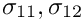and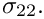Here is the implementation: The "wrapping" element inherits from the element type specified by the template parameter and calls its constructor (recall that element constructors are always argument-free!).

//=================start_wrapper==================================
/// Wrapper class for solid elements to modify their output
/// functions.
//================================================================
template <class ELEMENT>
class MySolidElement : public virtual ELEMENT
{
public:
/// Constructor: Call constructor of underlying element
MySolidElement() : ELEMENT() {};

We then overload the (virtual) output function so that the element outputs its shape, i.e. the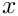and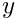coordinates of its plot points, and the three independent components of the second Piola Kirchhoff stress at those points. Other than that, the element behaves exactly as the underlying "wrapped" element.

void output(std::ostream &outfile, const unsigned &n_plot)
{
// Element dimension
unsigned el_dim = this->dim();
Vector<double> s(el_dim);
Vector<double> x(el_dim);
DenseMatrix<double> sigma(el_dim,el_dim);
switch(el_dim)
{
case 2:
outfile << "ZONE I=" << n_plot << ", J=" << n_plot << std::endl;
//Loop over element nodes
for(unsigned l2=0;l2<n_plot;l2++)
{
s = -1.0 + l2*2.0/(n_plot-1);
for(unsigned l1=0;l1<n_plot;l1++)
{
s = -1.0 + l1*2.0/(n_plot-1);
// Get Eulerian coordinates and stress
this->interpolated_x(s,x);
this->get_stress(s,sigma);
//Output the x,y,..
for(unsigned i=0;i<el_dim;i++)
{outfile << x[i] << " ";}
// Output stress
outfile << sigma(0,0) << " "
<< sigma(1,0) << " "
<< sigma(1,1) << " "
<< std::endl;
}
}
break;
default:
std::ostringstream error_message;
error_message << "Output for dim !=2 not implemented" << std::endl;
throw OomphLibError(error_message.str(),
OOMPH_CURRENT_FUNCTION,
OOMPH_EXCEPTION_LOCATION);
}
}
};

[Note: Since this element is only defined and used in a 2D driver code, there is little point in implementing the output for 1D or 3D elements. However, paranoid as we are, we check the dimension of the element and throw an error if it is wrong.]

Finally we declare that the FaceGeometry of the "wrapped" element is the same as that of the underlying element. This step is required to allow the automatic construction of SolidTractionElements in create_traction_elements().

//===========start_face_geometry==============================================
/// FaceGeometry of wrapped element is the same as the underlying element
//============================================================================
template<class ELEMENT>
class FaceGeometry<MySolidElement<ELEMENT> > :
public virtual FaceGeometry<ELEMENT>
{
public:
/// Constructor [this was only required explicitly
/// from gcc 4.5.2 onwards...]
FaceGeometry() : FaceGeometry<ELEMENT>() {}
};